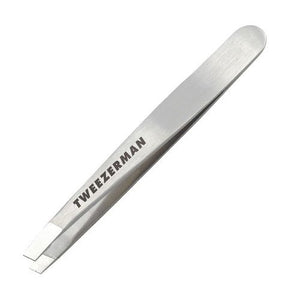# Tweezerman - Mini Slant Tweezer Stainless Steel

• \$34.29
• Save \$3.89

• T

• h

• i

• s

• m

• i

• n

• i

• t

• w

• e

• e

• z

• e

• r

• f

• i

• t

• s

• a

• n

• y

• t

• i

• n

• y

• p

• u

• r

• s

• e

• o

• r

• b

• u

• d

• g

• e

• t

• w

• i

• t

• h

• o

• u

• t

• s

• a

• c

• r

• i

• f

• i

• c

• i

• n

• g

• p

• r

• e

• c

• i

• s

• i

• o

• n

• Color : Classic Stainless

The perfect on the go tweezer, this smaller version of our award-winning Slant fits any tiny clutch or tiny budget. The ideal size for make-up and travel bags, our mini features the same perfect precision as its full size sister. In classic stainless steel finish.

every pages